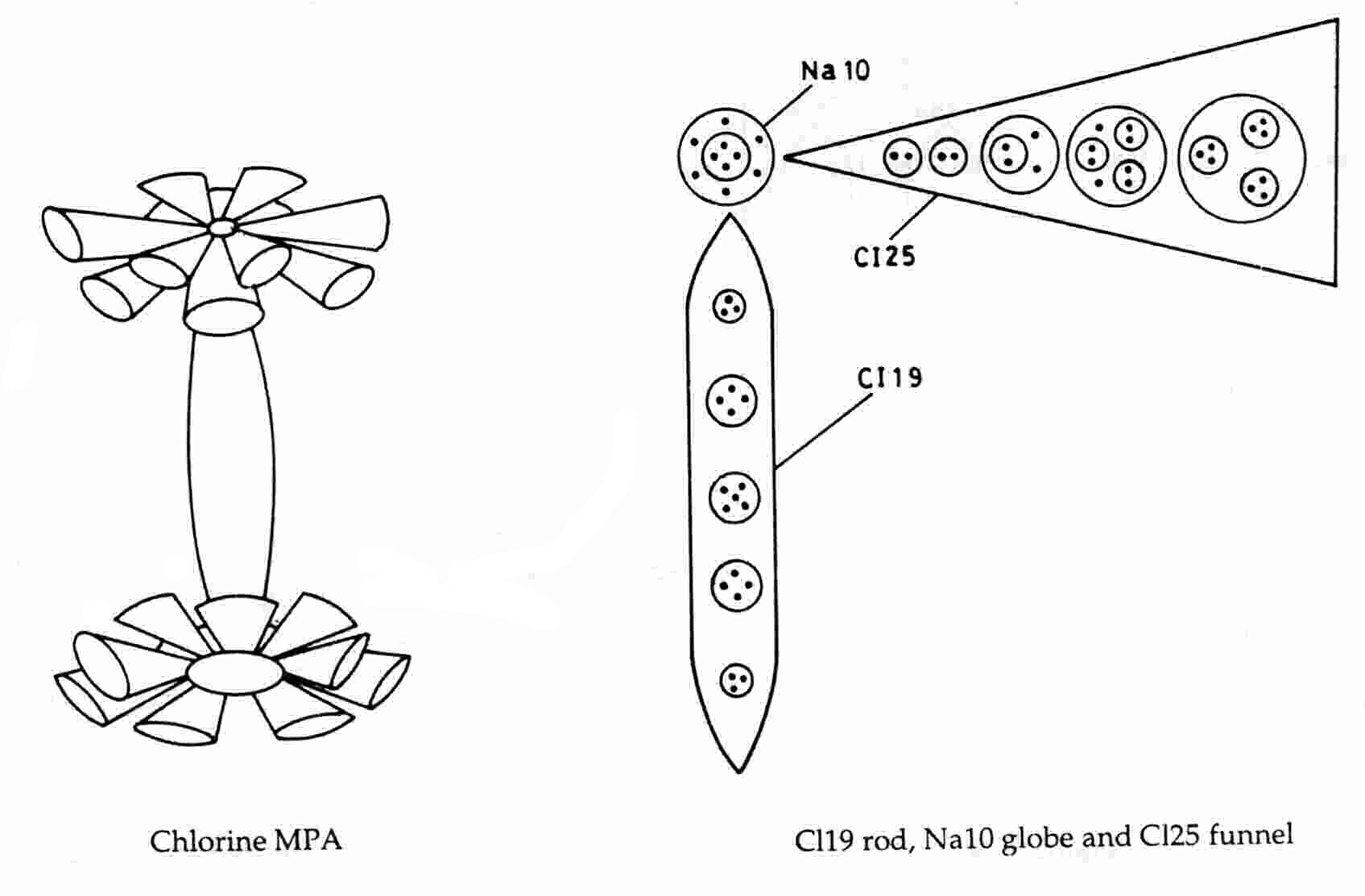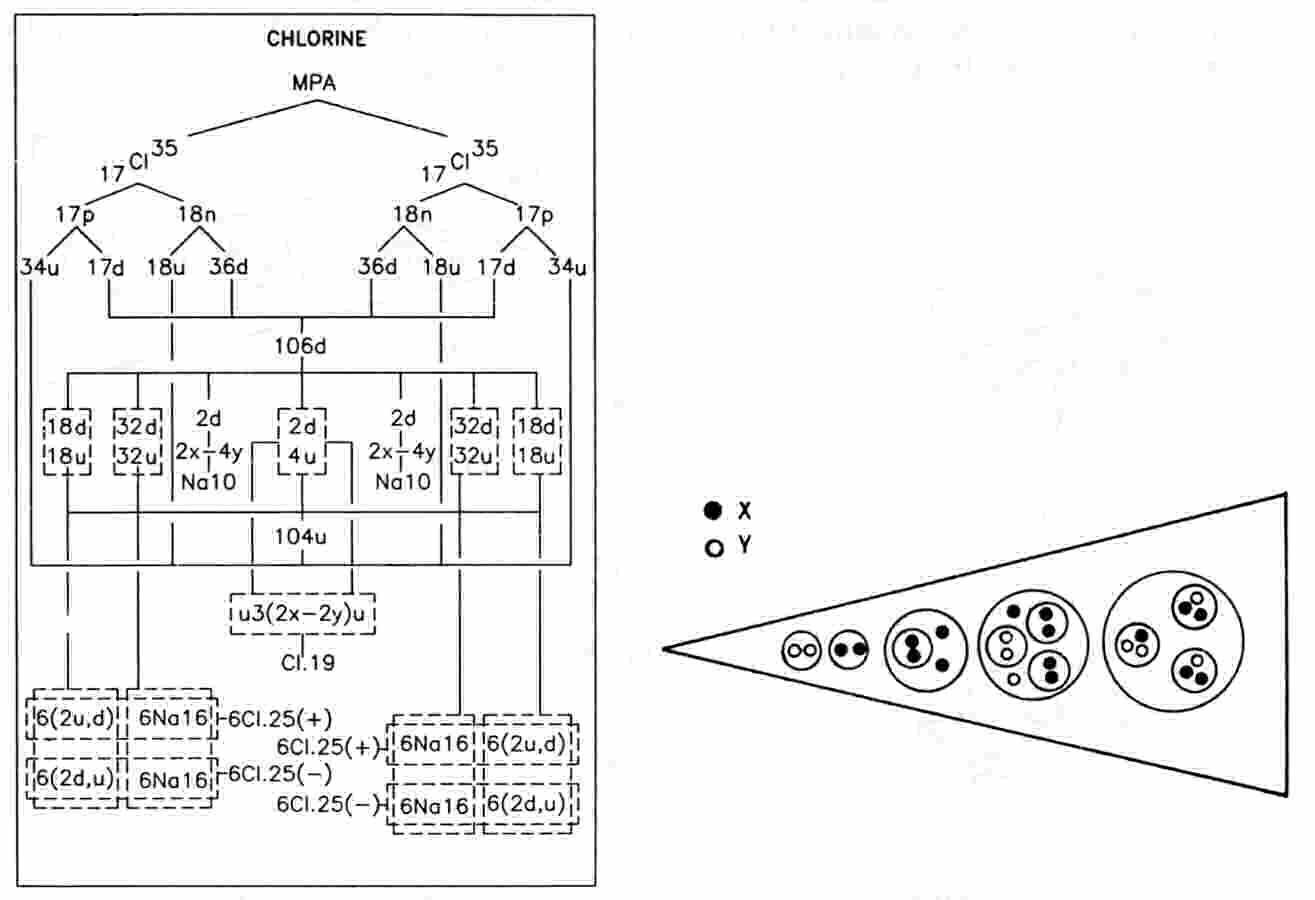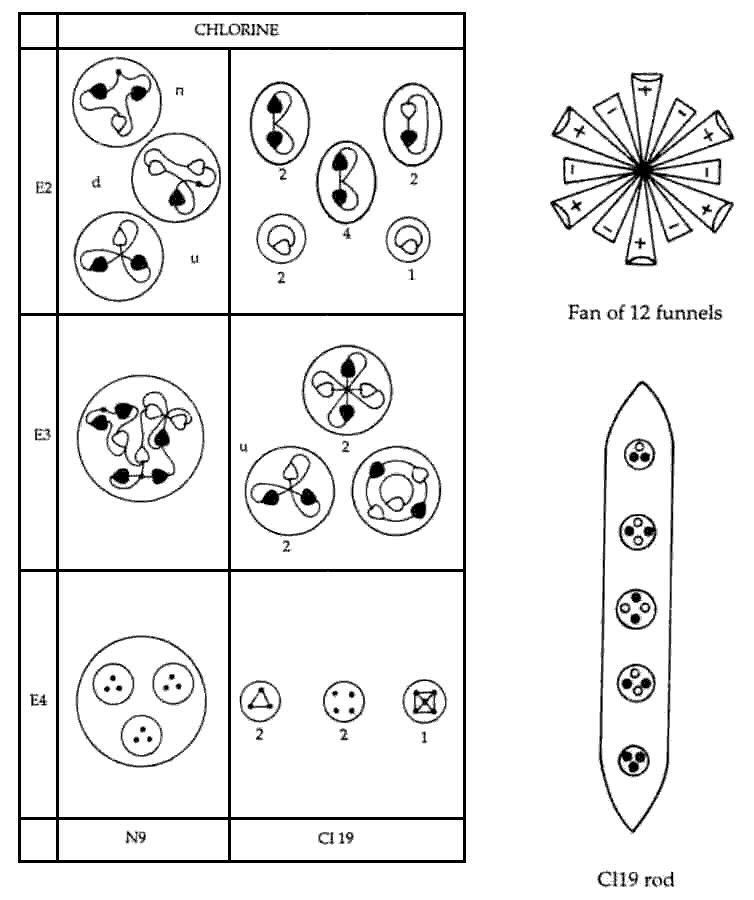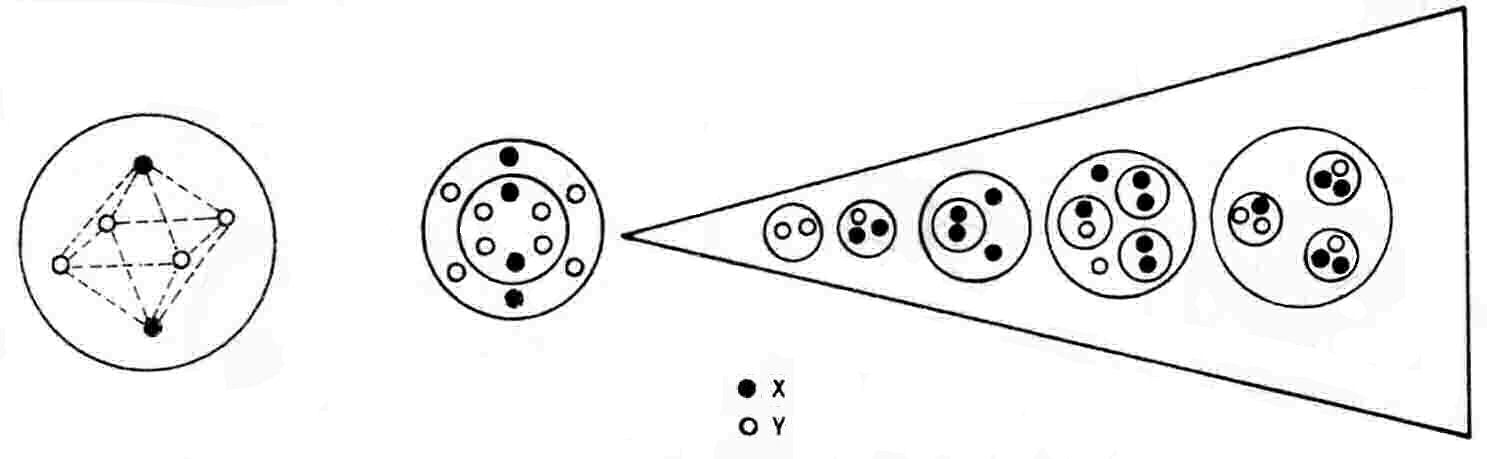The Chlorine MPAThe chlorine MPA is shaped like a dumb-bell. The central rod (CL19) consists of five small spheres containing three, four, five, four and three UPAs. At each end of the rod are Na10 globes from which radiate twelve funnels. A funnel contains a set of three hydrogen triplets (N9) in addition to the contents (Na16) of a funnel of the sodium MPA. There are twenty-five UPAs in a funnel, the set of particles being called a "Cl25 group."

Chlorine MPA = Cl19 + 2Na10 + 24Cl25.

The MPA is formed form two Cl35 nuclei, which provide 630 subquarks — nine fewer than the number of UPAs. Three hundred subquarks in fifty u quarks and fifty d quarks are equally distributed among a set of twelve funnels, twenty-five to a funnel. The analysis of the sodium MPA reveals that the subquarks in thirty-two u quarks and thirty-two d quarks are distributed amongst a set of twelve Na16 funnels. Each set of twelve Cl25 funnels contains the subquarks making up eighteen more u quarks and eighteen more d quarks.Each of six funnels contains an extra two u quarks and a d quark. Each of the other six funnels contains two extra d quarks and an extra u quark. These u-u-d and d-d-u bound states are, respectively, protons and neutrons (analysis of the nitrogen MPA in the author's book Extra-sensory Perception of Quarks identified an N9 group as a neutron; here the proton, its mirror state N9′, is also present). The disintegration diagram confirms that, for the funnel examined, the N9 breaks up at stage E2 into two (−) triplets (d quarks) and one (+) triplet (u quark).

Two types of Cl25 groups are predicted:

Cl25(+) = Na16 + u-u-d,

which has an electric charge of +53/9, and

Cl25(−) = Na16 + d-d-u,

which has a charge of −28/9. Six of each type are present in a set of twelve funnels at each end of the Cl19 rod, the two types alternating as shown in the diagram below. The first edition of Occult Chemistry confirms that six of the funnels are distinct from the other six, stating: "... the general form is that of the dumb-bell, the lower and upper parts each consisting of twelve funnels, six sloping upwards and six downwards."1

The Cl19 rod is made up of two u quarks, two 2X-2Y bound states and a central group of two X subquarks and two Y subquarks. The disintegration diagram confirms that two (+) triplets (u quarks) are released at stage E3 of the break-up of the Cl19 rod. It also indicates that the two groups of four UPAs break up into four (+) duads. This must be an error of observation, for these groups should have split up into two (+) duads (X-X) and two (−) duads (Y-Y). The reported group of five UPAs at the centre of the rod should be a group of four, that is, the Cl19 should contain eighteen UPAs. The subquarks in two d quarks make up an Na10 globe, which should contain six, not ten, UPAs (see the diagram below). En passim, it should be noted that Figure 7.104 in Extra-sensory Perception of Quarks depicts the corrected Na10, not the Cl19. The discrepancy of 9 between the 630 subquarks predicted to be in the MPA of chlorine and the counted population of 639 UPAs arises from overcounting by 1 for the Cl19 rod and by 4 for each Na10 globe. Evidence supporting this prediction is as follows: Besant & Leadbeater reported a slightly more complex form of the chlorine MPA in which each funnel has an extra UPA and each globe contains two octahedral arrays of UPAs, one inside the other:MPA = Cl19 + 2(Na10 + 2) + 24Cl26.

The MPA contains 667 UPAs. This is the MPA formed from two Cl37 nuclei, which provide 666 subquarks. If the Cl19 rod actually contains eighteen UPAs, the counted and predicted populations would be equal. This is highly significant and powerful confirmation of the prediction of an overcounting of the UPAs in the Cl19 rod by one. Besant & Leadbeater noted that "The isotope is less common than the normal variety of Chlorine."2 This is consistent with the MPA being formed from two Cl37 nuclei because the Cl35 nuclide has a relative abundance of 75.53%, whereas the Cl37 nuclide has an abundance of 24.47%.

The difference between the MPAs of the two chlorine isotopes should amount to sixteen X subquarks and twenty Y subquarks contributed by four extra neutrons in the pair of Cl37 nuclei. These permit the extra UPA to be an X subquark in each of twelve funnels and a Y subquark in each of the twelve other funnels, the innermost X-X duad becoming a triplet (u quark) by the addition of a Y subquark and the innermost Y-Y duad becoming a triplet (d quark) by the addition of an X subquark. Two X subquarks and four Y subquarks should therefore be added to each Na10 globe, which already contains two X and four Y subquarks, according to the analysis of the sodium MPA, i.e., each globe should contain four X subquarks and eight Y subquarks. In his search for an isotope of chlorine in sea water, Leadbeater said3 that the twelve UPAs in each globe were arranged in two octahedral clusters, one inside the other. Given that a bound state of two X subquarks and four Y subquarks is octahedral, the presence of a second octahedral array is consistent with the extra subquarks also consisting of two X subquarks and four Y subquarks — in agreement with prediction.

References

1. Occult Chemistry (1st ed.), p. 35.

2. Occult Chemistry (3rd ed.), p. 66.

3. Ibid, p. 351.

Home